# Civil Engineering - UPSC Civil Service Exam Questions

11.

The stress distribution at a depth beneath a loaded area is determined using Newmark's influence chart which indicates an influence value of 0.005. The number of segments covered by the loaded area in the chart is 20 and the intensity of loading on the area is 10 T/m2. The intensity of stress distribution at that depth is

 A. 1 T/m2 B. 2 T/m2 C. 5 T/m2 D. 10 T/m2

Explanation:

No answer description available for this question. Let us discuss.

12.

A two-hinged semicircular arch of radius 'R' carries a concentrated load 'W' at the crown. The horizontal thrust is

 A.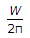B.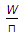C.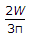D.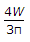Explanation:

No answer description available for this question. Let us discuss.

13.

The adjoining figures show funicular diagram and polar diagram for a beam subjected to vertical forces. x is the distance of the pole from the vector line. If x is doubled, then the ordinate in the funicular diagram will be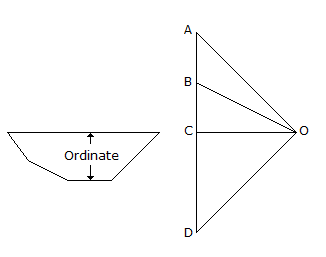A. doubled B. halved C. unaffected D. tripled

Explanation:

No answer description available for this question. Let us discuss.

14.

In a cohesionless soil deposit having a unit weight of 1.5 t/m3and an angle of internal friction of 30° the active and passivelateral earth pressure intensities (in t/m2) at a depth of 10 m will, respectively, be

 A. 15 and B. 5 and 15 C. 10 and 20 D. 20 and 10

Explanation:

No answer description available for this question. Let us discuss.

15.

A three-hinged loaded semicircular arch ACB is shown in the figure given. What is the shearing force at the hinge C ?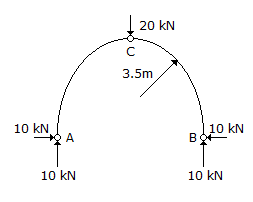A. 20 kN B. 20 2 kN C. 10 kN D. 10 2 kN